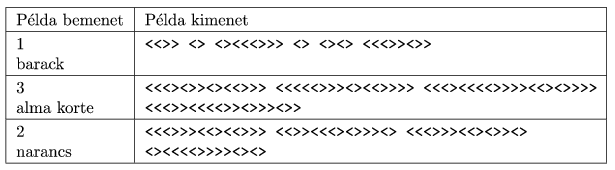Mathematical and Physical Journal
for High Schools
Issued by the MATFUND Foundation
 Already signed up? New to KöMaL?

#Problem S. 65. (October 2011)

S. 65. Even in the ancient Aztec empire they found it necessary to encrypt messages issued by the rulers. According to the famous archeologist, Prof. Stoory, codes were based on the two symbols of Ometeotl, the lord of the duality: the < symbol representing masculine force, and the symbol > representing feminine affection. It was only allowed to create strings that obeyed the following two rules:

-- according to the principle of balance, each string should contain the same number of < and > symbols,

-- reading from left to right, up to any symbol, the number of masculine symbols should be greater than or equal to that of the feminine symbols. In other words, symbols representing force and activity precede symbols representing affection and passivity.

Strings of the above type can be sorted according to their length and the position of the < symbols:

(1) shorter strings are listed first, and

(2) if two strings have the same length, lexicographic ordering is used: the order is determined by the first character, but if they are the same, then by the second character, and so on.

For example, the order of the strings of length at most 6 is the following:

<>

<<>>

<><>

<<<>>>

<<><>>

<<>><>

<><<>>

<><><>

Therefore we have an (infinite) ordered list R of symbols < and >. Then the steps for creating the code by the Aztecs were the following:

(1) The letters of the language (for the sake of simplicity, we now use the lowercase English alphabet) together with the space character are represented by the digits of the number system in base 27 (,,, . . . ,).

(2) The message is split into blocks of length b (if the length of the message is not a multiple of b, the minimal number of spaces is added to the right to make it divisible).

(3) Then blocks are interpreted as integers in base 27.

(4) Finally they select the corresponding <, > symbol strings from the above list R (the number of the string in R is the same as the number they got in Step 3), space separators are inserted between each block, and these strings are concatenated.

Your program should be able to encode and decode messages from the ruler.

The first line of the input file contains the block length b (1b12). The second line contains a character string to be encoded or decoded. Except for the spaces, a string to be encoded contains only lowercase letters of the English alphabet, while strings to be decoded only the < and > characters. We may assume that the input does not begin with a space.

The only line of the output file should contain the result of the encoding or decoding.

In the example, Példa bemenet'' is the sample input, while Példa kimenet'' is the sample output. We remark that in the example there may be line breaks instead of certain space characters.(10 pont)

Deadline expired on November 10, 2011.

Sorry, the solution is available only in Hungarian. Google translation

A feladatot dinamikus programozással lehetett hatékonyan megoldani. Mintamegoldásként Adrián Patrik, és Marussy Kristóf megoldásait közöljük.

A két megoldás hasonló, viszont utóbbiban egy formálisabb, részletesebb leírás található.

### Statistics:

 11 students sent a solution. 10 points: Adrián Patrik, Havasi 0 Márton, Marussy Kristóf, Nagy Róbert, Strenner Péter, Szabó 262 Lóránt, Szilágyi Dániel. 9 points: Szabó 928 Attila. 7 points: 1 student. 5 points: 1 student. 3 points: 1 student.

Problems in Information Technology of KöMaL, October 2011